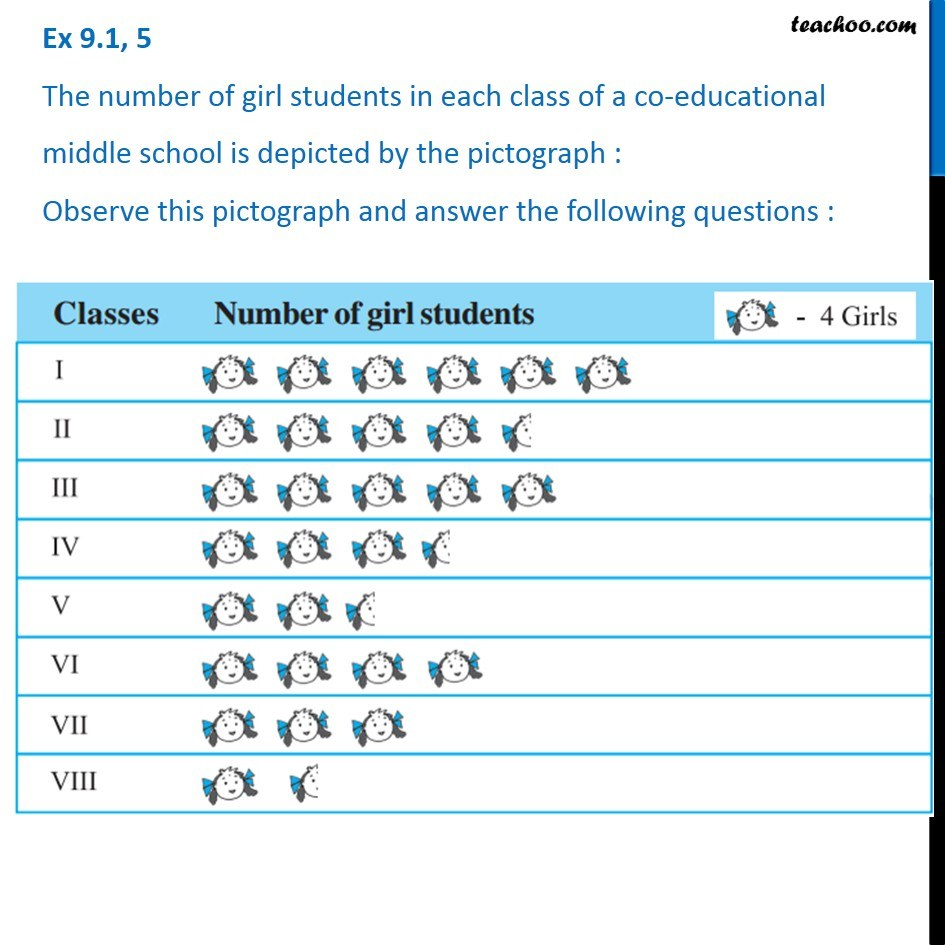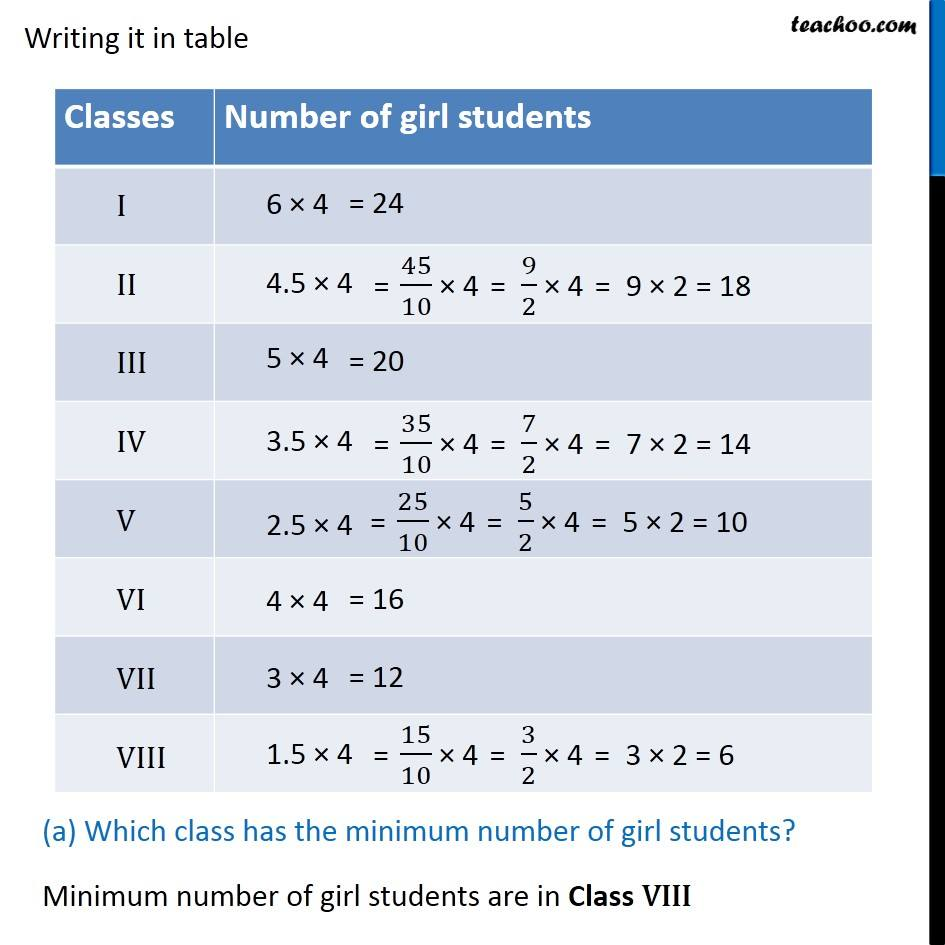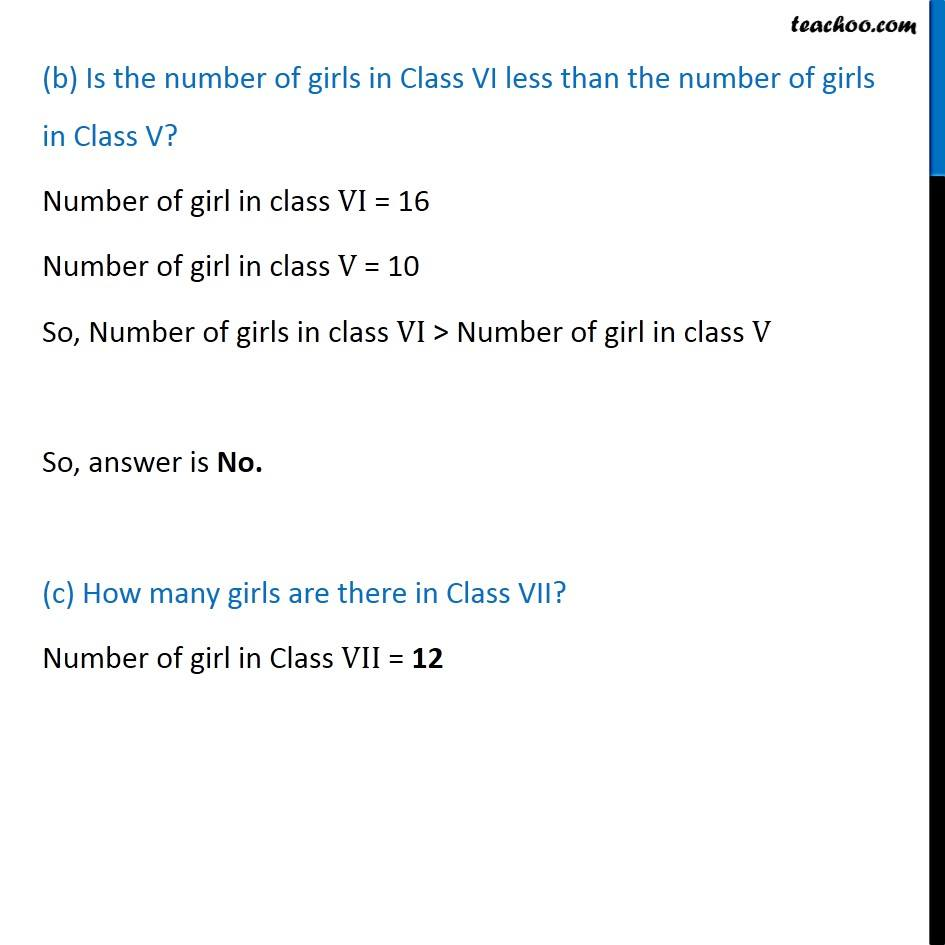1. Chapter 9 Class 6 Data Handling
2. Serial order wise
3. Ex 9.1

Transcript

Ex 9.1, 5 The number of girl students in each class of a co-educational middle school is depicted by the pictograph : Observe this pictograph and answer the following questions : Writing it in table (a) Which class has the minimum number of girl students? Minimum number of girl students are in Class VIII (b) Is the number of girls in Class VI less than the number of girls in Class V? Number of girl in class VI = 16 Number of girl in class V = 10 So, Number of girls in class VI > Number of girl in class V So, answer is No. (c) How many girls are there in Class VII? Number of girl in Class VII = 12

Ex 9.1

Chapter 9 Class 6 Data Handling
Serial order wise